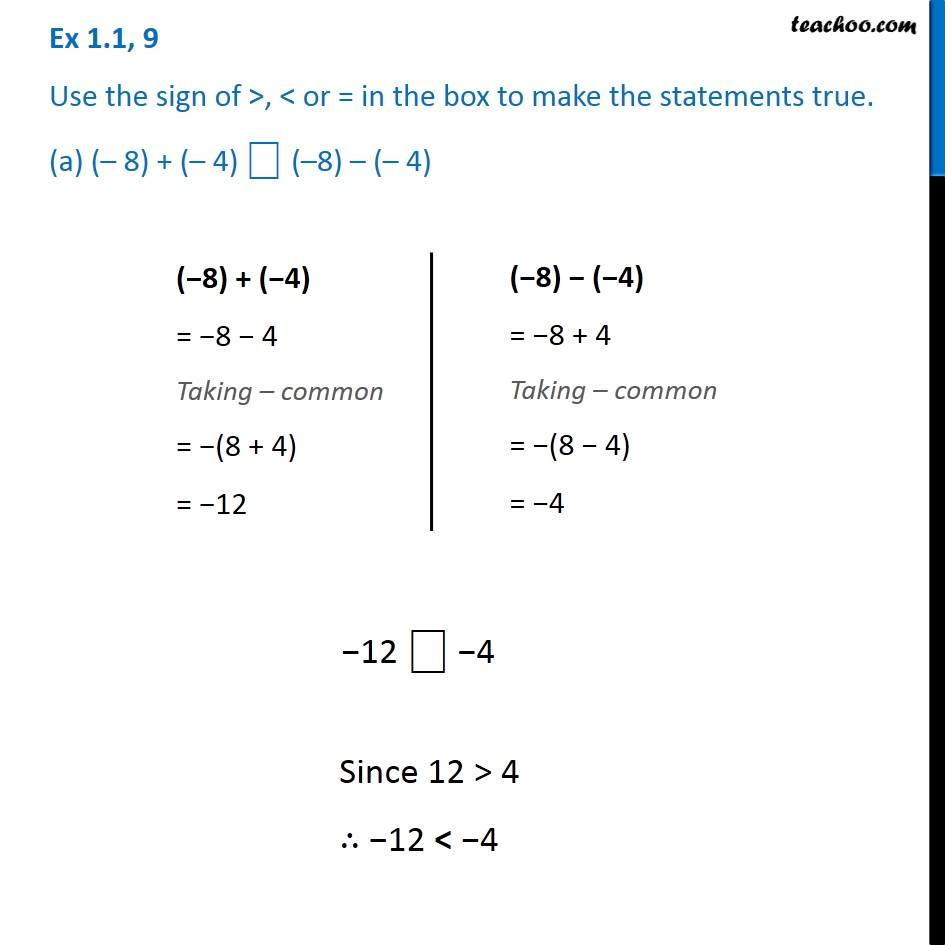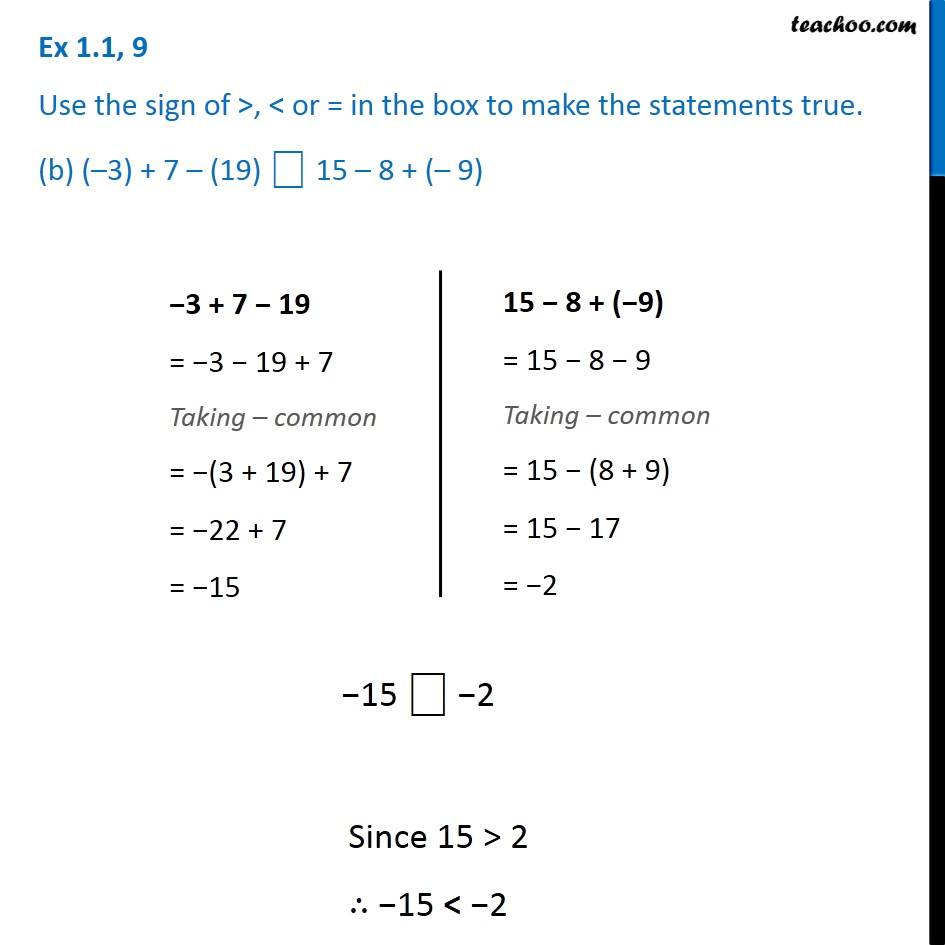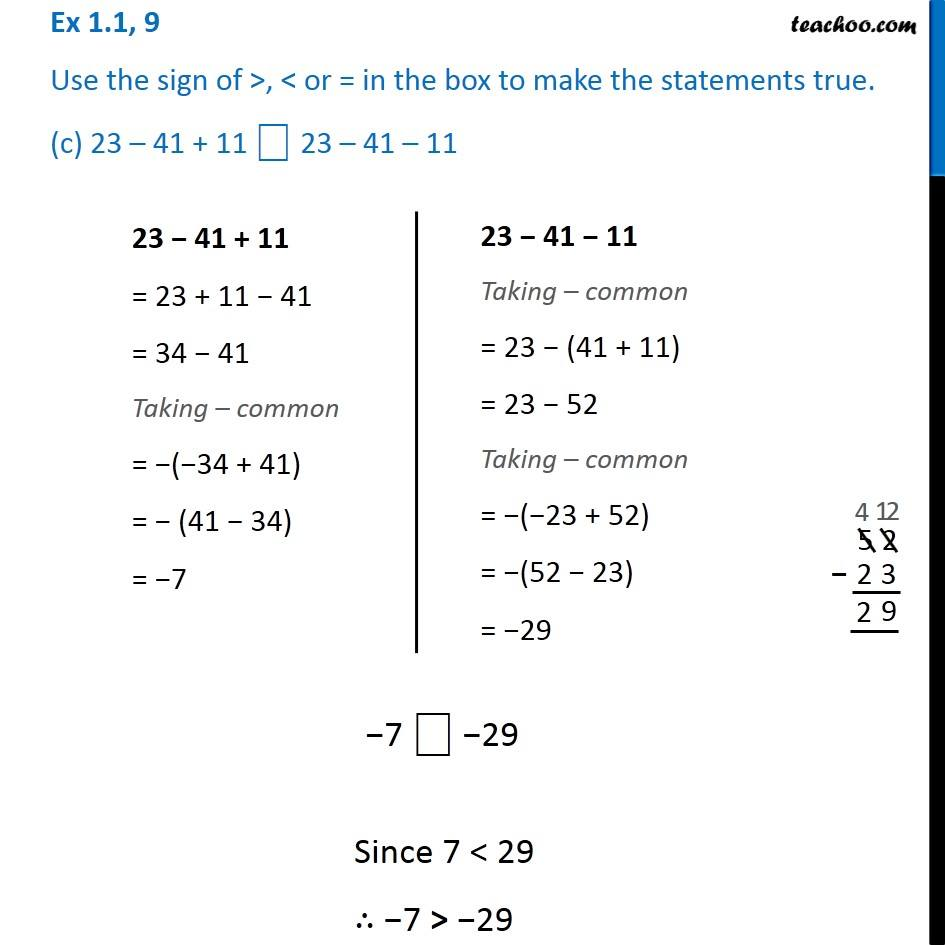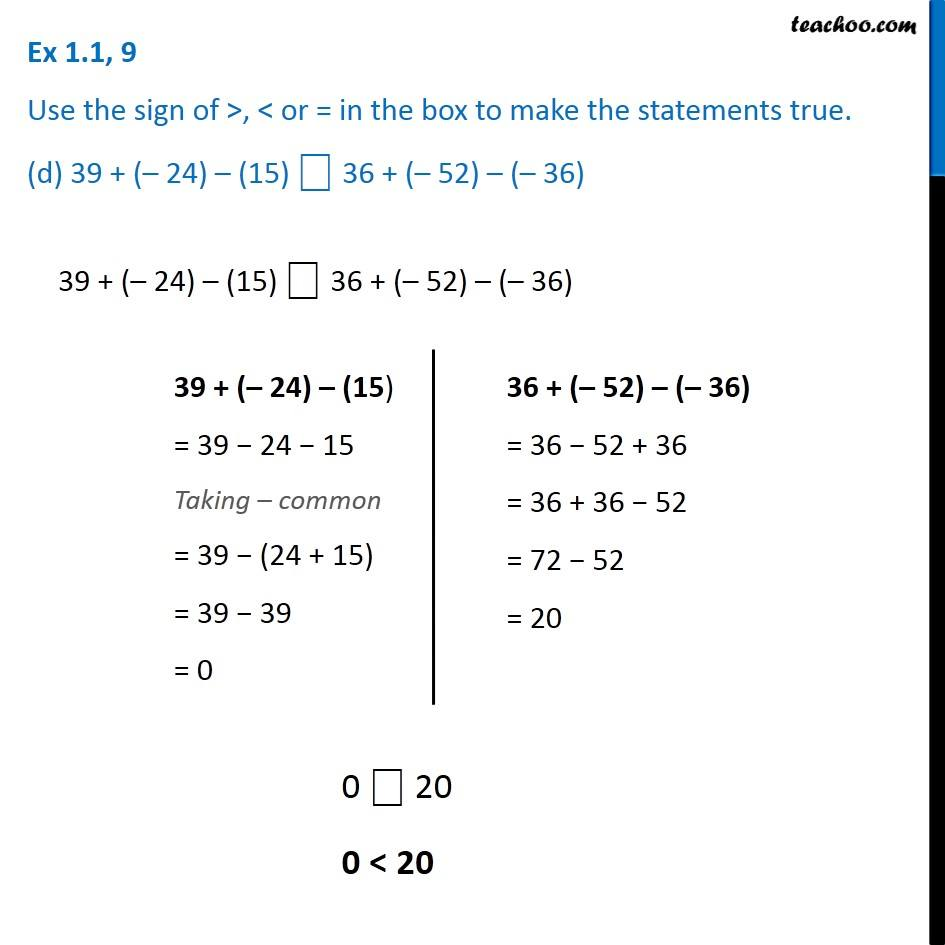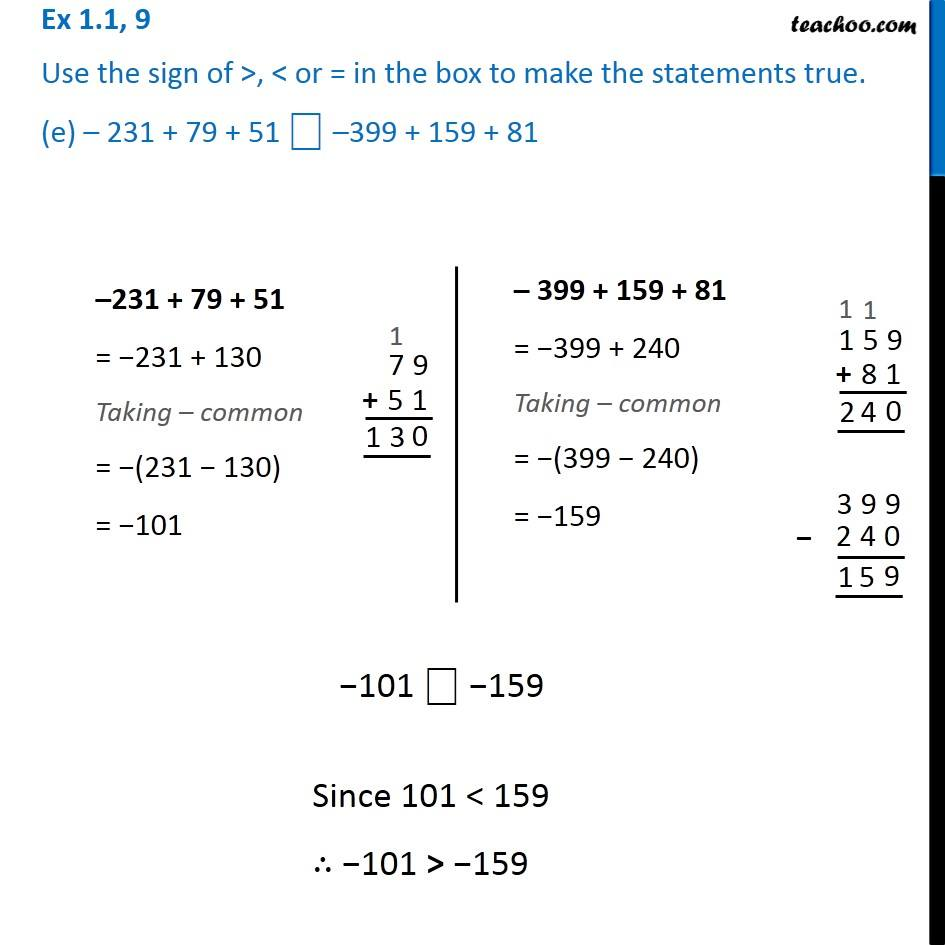Subscribe to our Youtube Channel - https://you.tube/teachoo

1. Chapter 1 Class 7 Integers
2. Concept wise
3. Additon and subtraction of Integers

Transcript

Ex 1.1, 9 Use the sign of >, < or = in the box to make the statements true. (a) (– 8) + (– 4) ⎕ (–8) – (– 4)(−8) + (−4) = −8 − 4 Taking – common = −(8 + 4) = −12 (−8) − (−4) = −8 + 4 Taking – common = −(8 − 4) = −4 −12 ⎕ −4 −12 ⎕ −4 Ex 1.1, 9 Use the sign of >, < or = in the box to make the statements true. (b) (–3) + 7 – (19) ⎕ 15 – 8 + (– 9)−3 + 7 − 19 = −3 − 19 + 7 Taking – common = −(3 + 19) + 7 = −22 + 7 = −15 15 − 8 + (−9) = 15 − 8 − 9 Taking – common = 15 − (8 + 9) = 15 − 17 = −2 −15 ⎕ −2 Since 15 > 2 ∴ −15 < −2 Ex 1.1, 9 Use the sign of >, < or = in the box to make the statements true. (c) 23 – 41 + 11 ⎕ 23 – 41 – 1123 − 41 + 11 = 23 + 11 − 41 = 34 − 41 Taking – common = −(−34 + 41) = − (41 − 34) = −7 23 − 41 − 11 Taking – common = 23 − (41 + 11) = 23 − 52 Taking – common = −(−23 + 52) = −(52 − 23) = −29 7 ⎕ −29 Since 7 < 29 ∴ −7 > −29 Ex 1.1, 9 Use the sign of >, < or = in the box to make the statements true. (d) 39 + (– 24) – (15) ⎕ 36 + (– 52) – (– 36)39 + (– 24) – (15) ⎕ 36 + (– 52) – (– 36) 39 + (– 24) – (15) = 39 − 24 − 15 Taking – common = 39 − (24 + 15) = 39 − 39 = 0 36 + (– 52) – (– 36) = 36 − 52 + 36 = 36 + 36 − 52 = 72 − 52 = 20 0 ⎕ 20 0 < 20 Ex 1.1, 9 Use the sign of >, < or = in the box to make the statements true. (e) – 231 + 79 + 51 ⎕ –399 + 159 + 8139 + (– 24) – (15) ⎕ 36 + (– 52) – (– 36) 39 + (– 24) – (15) = 39 − 24 − 15 Taking – common = 39 − (24 + 15) = 39 − 39 = 0 36 + (– 52) – (– 36) = 36 − 52 + 36 = 36 + 36 − 52 = 72 − 52 = 20 0 ⎕ 20 0 < 20 Ex 1.1, 9 Use the sign of >, < or = in the box to make the statements true. (e) – 231 + 79 + 51 ⎕ –399 + 159 + 81–231 + 79 + 51 = −231 + 130 Taking – common = −(231 − 130) = −101 – 399 + 159 + 81 = −399 + 240 Taking – common = −(399 − 240) = −159 −101 ⎕ −159 −101 ⎕ −159